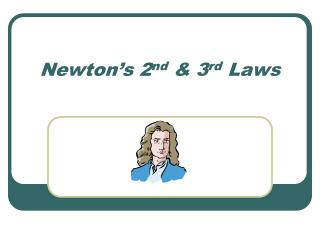DownloadDownload PresentationNewton’s 2 nd & 3 rd Laws

# Newton’s 2 nd & 3 rd Laws

Download Presentation## Newton’s 2 nd & 3 rd Laws

- - - - - - - - - - - - - - - - - - - - - - - - - - - E N D - - - - - - - - - - - - - - - - - - - - - - - - - - -
##### Presentation Transcript

1. Newton’s 2nd & 3rd Laws

2. A couple things to keep straight • Mass = the amount of “stuff” something is made of. • Weight = the measure of the force of gravity on an object.

3. A couple of things to remember • You can use Newton’s 2nd Law (F=ma) to solve for weight. • Weight = force of gravity acting on a specific object. (acceleration due to gravity is 9.8m/s2 ) therefore…

4. How to solve for weight • Fnet = m x a (2nd Law) • Weight = mass of object x acc. due to gravity • F(grav) = m x acc (due to “g”) • Weight = m x acc (due to “g”) so: F(grav) = weight = m x g

5. Standard Units of Force • Force measured in Newtons (N) • 1 N = 1 kg x m/s2 • 1 Kg weighs approximately 2.2 lbs

6. A question to make you think??? • Is the force of gravity the same for all objects on Earth? Answer… NO!!! Due to different mass, however acceleration is the same for all objects! Think about the force an object exerts on Earth when it lands.

7. A couple things about Newton’s 3rd Law • Forces always occur in pairs • “For every action there is an equal & opposite reaction” Fa on b = -Fb on a Ex. Car driving down the road. What are the forces?

8. A couple things about Newton’s 3rd Law (cont.) What forces are acting on the car? Fg (down) / FN (up) Force of friction (road on car) / Force of friction (car on road)

9. A couple things about Newton’s 3rd Law (cont.) • Drop a softball (mass 0.18Kg) ? What is the force on Earth due to the ball ? What is the Earth’s acceleration (mass of Earth = 6.0 x 1024 Kg) hint: use Fg = mg

10. Softball Answer • F(grav) = (0.18 kg) x (-9.8 m/s2) F = -1.8 N (therefore, force on ball from Earth = 1.8 • Acceleration = 1.8 N = (6.0E24)a 3.1E-25 m/s2